• 哈希表设计
千次阅读
2022-05-02 19:28:23

[问题描述]

针对某个集体中人名设计一个哈希表，使得平均查找长度不超过2，并完成相应的建表和查表程序。

[基本要求]

假设人名为中国人姓名的汉语拼音形式。待填入哈希表的人名共有30个，取平均查找长度的上限为2。哈希函数用除留余数法构造，用线性探测再散列法或链地址法处理冲突。

[测试数据]

取自己周围较熟悉的30个人名。

[选作内容]

（1） 从教科书上介绍的集中哈希函数构造方法中选出适用者并设计几个不同的哈希函数，比较他们的地址冲突率（可以用更大的名字集合作实验）。
（2） 研究这30个人名的特点，努力找一个哈希函数，使得对于不同的拼音名一定不发生地址冲突。
（3）在哈希函数确定的前提下尝试各种不同处理冲突的方法，考察平均查找长度的变化和造好的哈希表中关键字的聚集性。

[代码实现]

/*
0!='0'
*/
#include<queue>
#include<stdio.h>
#include<string.h>
#include<stdlib.h>
#include<algorithm>
using namespace std;
#define scnaf scanf
#define ll long long
const int N=1000;
int n=30,l=0x3f3f3f3f,r=0,flag,cut=0;
char s[N],x;
int hashnum1(int k)//1.hash函数|取余法
{
r=max(r,k%31+1);
l=min(l,k%31+1);
return k%31+1;
}
int hashnum2(int k)//2.hash函数|数字分析法
{
int p,i=1,t=0;
while(k)
{
p=k%10;
if(i<3)
t+=p*i++;
else t+=p*i--;
k/=10;
}
r=max(r,t);//
l=min(l,t);
return t;//
}
int hashnum3(char asc[])//直接定址法，该法针对所给30个人名样本不产生冲突
{
int p=0,i,j;
for(i=0,j=0;asc[i];i++,j++)
p+=asc[i]*i;
p=(p/1000*10+p/100%10)*(p/10%10*10+p%10);
p/=10;
r=max(r,p);
l=min(l,p);
return p;
}
int slove(char asc[],int f)//分配哈希函数
{
int ans=0,i;
for(i=0;asc[i];i++)
ans+=asc[i];
switch(f)
{
case 0:return ans;
case 1:return hashnum1(ans);
case 2:return hashnum2(ans);
case 3:return hashnum3(asc);
}
}
/*****以下链地址法解决冲突******/
struct Node
{
char key;//名字
struct Node *next;
};
struct Hash
{
int sum;//冲突次数
struct Node *data;//结点
}h[N];
void init()//malloc->free
{
struct Node *q,*p;
for(int i=l;i<=r;i++)
{
h[i].sum=0;
p=h[i].data;
while(p!=NULL)
{
q=(*p).next;
free(p);
p=q;
}
}
for(int i=l;i<=r;i++)
h[i].data=NULL;
l=0x3f3f3f3f,r=0;
}
void inserthash(int k,char asc[])//插入
{
struct Node *q;
q=(struct Node *)malloc(sizeof(struct Node));
strcpy((*q).key,asc);
(*q).next=h[k].data;
h[k].data=q;
h[k].sum++;
}
int searchhash(int k,char asc[])//查找
{
struct Node *p=h[k].data;
int cut=0;
while(p!=NULL)
{
cut++;
if(strcmp(asc,(*p).key)==0)
return cut;
p=(*p).next;
}
return 0;
}
void printff()//打印哈希表
{
printf("哈希表如下所示:\n");
for(int i=l;i<=r;i++)
{
printf("%d",i);
if(h[i].data!=NULL)
{
struct Node *p=h[i].data;
while(p!=NULL)
{
printf(" ->%s",(*p).key);
p=(*p).next;
}
}
printf("\n");
}
}
void chongtu()//平均查找长度
{
int i,k=0;
for(i=1;i<=n;i++)
k+=searchhash(slove(s[i],flag),s[i]);
switch(flag)
{
case 1:
{
printf("您选择的是取余法,平均查找长度为:%.2lf\n",k*1.0/n);
return ;
}
case 2:
{
printf("您选择的是数字分析法,平均查找长度为:%.2lf\n",k*1.0/n);
return ;
}
case 3:
{
printf("您选择的是直接定址法,平均查找长度为:%.2lf\n",k*1.0/n);
}
}
}
/**********************************/
/*****以下开放地址法解决冲突******/
struct ss
{
char name;
int length;
int emp;
}H[N];
void init2()//清零
{
memset(H,0,sizeof(H));
l=0x3f3f3f3f,r=0;
}
void inserthash2(int k,char asc[])//插入
{
int cnt=1,p=1;
while(H[k].emp)
{
if(cnt&1)
k=(k+p*p)%r;
else k=(k-p*p)%r,p++;
cnt++;
}
strcpy(H[k].name,asc);
H[k].length=cnt;
H[k].emp=1;
}
int searchhash2(int k,char asc[])//查找
{
int cnt=0,p=1;
while(H[k].emp)
{
cnt++;
if(strcmp(asc,H[k].name)==0)
return cnt;
if(cnt&1)
k=(k+p*p)%r;
else k=(k-p*p)%r,p++;
}
if(!H[k].emp)
return 0;
}
void printff2()//打印哈希表
{
printf("哈希表如下所示:\n");
for(int i=l;i<=r;i++)
{
printf("%d",i);
if(H[i].emp)
printf(" ->%s %d",H[i].name,H[i].length);
printf("\n");
}
}
void chongtu2()//平均查找长度
{
int i,k=0;
for(i=1;i<=n;i++)
k+=searchhash2(slove(s[i],flag),s[i]);
switch(flag)
{
case 1:
{
printf("您选择的是取余法,平均查找长度为:%.2lf\n",k*1.0/n);
return ;
}
case 2:
{
printf("您选择的是数字分析法,平均查找长度为:%.2lf\n",k*1.0/n);
return ;
}
case 3:
{
printf("您选择的是直接定址法,平均查找长度为:%.2lf\n",k*1.0/n);
}
}
}
/*********************************/

int main()
{
int i,j,k,p,v;
printf("请输入姓名:\n");
for(i=1;i<=n;i++)
scnaf("%s",s[i]);
printf("\n请选择冲入处理方式:\n 1.链地址法\n 2.开放定址法\n");
scanf("%d",&v);
if(v&1)
{
printf("\n您选择的是链地址法\n");
printf("\n请选择哈希函数:\n 1.取余法\n 2.数字分析法\n 3.直接定址法\n");
scanf("%d",&flag);
init();
for(i=1;i<=n;i++)
{
k=slove(s[i],flag);
inserthash(k,s[i]);
}
switch(flag)
{
case 1:printf("\n您选择的是取余法\n");break;
case 2:printf("\n您选择的是数字分析法\n");break;
case 3:printf("\n您选择的是直接定址法\n");break;
}
while(1)
{
printf("\n请输入:\n 0.退出\n 1.插入名字\n 2.查找名字\n 3.打印哈希表\n 4.平均查找长度\n");
scnaf("%d",&p);
if(!p)
{
init();
break;
}
switch(p)
{
case 1:
{
printf("\n请输入您要添加的名字:\n");
scnaf("%s",s[++n]);
k=slove(s[n],flag);
inserthash(k,s[n]);
break;
}
case 2:
{
printf("\n请输入您要查找的名字:\n");
scnaf("%s",x);
k=slove(x,flag);
j=searchhash(k,x);
if(!j)
printf("\n查找失败,请重新输入\n");
else printf("\n查找成功,查找长度为:%d\n",j);
break;
}
case 3:printff();break;
case 4:chongtu();break;
}
}
}
else
{
printf("\n您选择的是开放定址法\n");
printf("\n请选择哈希函数:\n 1.取余法\n 2.数字分析法\n 3.直接定址法\n");
scanf("%d",&flag);
init2();
for(i=1;i<=n;i++)
{
k=slove(s[i],flag);
inserthash2(k,s[i]);
}
switch(flag)
{
case 1:printf("\n您选择的是取余法\n");break;
case 2:printf("\n您选择的是数字分析法\n");break;
case 3:printf("\n您选择的是直接定址法\n");break;
}
while(1)
{
printf("\n请输入:\n 0.退出\n 1.插入名字\n 2.查找名字\n 3.打印哈希表\n 4.平均查找长度\n");
scnaf("%d",&p);
if(!p)
{
init2();
break;
}
switch(p)
{
case 1:
{
printf("\n请输入您要添加的名字:\n");
scnaf("%s",s[++n]);
k=slove(s[n],flag);
inserthash2(k,s[n]);
break;
}
case 2:
{
printf("\n请输入您要查找的名字:\n");
scnaf("%s",x);
k=slove(x,flag);
j=searchhash2(k,x);
if(!j)
printf("\n查找失败,请重新输入\n");
else printf("\n查找成功,查找长度为:%d\n",j);
break;
}
case 3:printff2();break;
case 4:chongtu2();break;
}
}
}
return 0;
}
/*
int main()
{
char v;
int a;
for(int i=1;i<=n;i++)
scnaf("%s",v[i]),a[i]=slove(v[i],1);
sort(a+1,a+n+1);
for(int i=1;i<=n;i++)
printf("%d\n",a[i]);
return 0;
}


/
//29|22-3 31|22-2
/

700~140
yangxinyi
lihaoyu
xuhaidong
songzongzui
wangzongyao
zhangwenqi
liudengtian
huoyuqing
fangyuanyuan
wangweiyi
wangyan
zhoumeichen
chenshuai
hanmeng
liyaran
yuxiaolei
lujiale
shichenghong
liuwanyou
chaiwenli
wanghaozhi
zhaobowen
gaoruiying
lijinfang
sunpeng
sunshanpeng
wanghongyang
xinghaora
qinyichen
xiuyuhang
*/数据结构 散列表
更多相关内容
• 哈希表设计程序设计+数据结构实验报告 1.1 针对某个集体中的人名设计一个哈希表，使得平均查找长度不超过R，完成相应的建立和查表程序. 1.2 人名为汉语拼音形式，最长不超过18个字符(如：庄双双 zhuangshuangshuang)...哈希表设计
• 针对某个集体（比如你所在的班级）中的“人名”设计一个哈希表，完成相应的建表和查表程序。 【基本要求】 假设人名为中国人姓名的汉语拼音形式。带填入哈希表的人名共有30个。哈希函数用除留余数法构造，用线性...哈希表设计
• 哈希表表长为20，用除留余数法构造一个哈希函数，以开放地址法中的线性探测再散列法作为解决冲突的方法，编程实现哈希表查找、插入和建立算法。
• 功能描述：针对自己的班同学名单设计一个哈希表，使得平均查找长度不超过2，完成相应的建表和查表程序。 设计要求：假设人名为中国姓名的汉语拼音形式,哈希函数用除留余数法构造，用链表法处理冲突。
• 哈希表设计，问题描述：针对某个集体（比如你所在的班级）中的“人名”设计一个哈希表，使得平均查找长度不超过r，完成相应的建表和查表程序。
• 哈希表课程设计数据结构实验报告——哈希表设计 针对某个集体中的人名设计一个哈希表，使得平均查找长度不超过R，完成相应的建立和查表程序. 1.2 人名为汉语拼音形式，最长不超过18个字符(如：庄双双 ...哈希表
• 山东科技大学学生课程设计 实习6哈希表设计 需求分析 1. 问题描述 针对某个集体比如你所在的班级中的人名设计一个哈希表使得平均查找长度均不超过R完成相应的建表和查表顺序 2. 基本要求 假设人名为中国人姓名的汉语...
• 实验六：存储管理、查找和排序 题目：哈希表设计 班级： 姓名： 学号： 1. 问题描述 针对某个集体（比如你所在的班级）中的"人名"设计一个哈希表，使得平均查找长度 均不超过R，完成相应的建表和查表顺序。 2. 基本...
• 利用哈希表设计快速电话号码查询系统 请你为自己手机的电话簿以电话号码作为关键字建立哈希表，然后依据电话号码进行哈希查找，并采用合适的冲突处理方法处理冲突。查找成功显示姓名与号码，查找失败则进行插入。...c语言 java
• 针对某个集体（比如你所在班级）中的“人名”设计一个哈希表，使得平均查找长度不超过R，完成相应的建表和查表程序。哈希表
• 针对某集合中的“人名”设计并实现一个哈希表。 任务要求：针对姓名信息进行初始化哈希表，可以进行显示哈希表，查找元素。 设计思想：哈希函数用除留余数法构造，用线性探测再散列处理冲突。 设人名为中国人姓名...
• C语言设计哈希表实现图书查找系统，完成相应的建表和查表程序。从键盘输入各图书相关信息，分别以图书编号为关键字建立散列表。待填入哈希表的书号至少30个；构造合适的哈希函数。 1） 记录由外部输入。 2） 将生成...
• 针对某个集体中人名设计一个哈希表，使得平均查找长度不超过R，并完成相应的建表和查表程序。 基本要求 假设人名为中国人姓名的汉语拼音形式。待填入哈希表的人名共有30个，取平均查找长度的上限为2。哈希函数用除...
• 石家庄铁道大学 刘立嘉 算法与数据结构 实验五 哈希表设计
• 1. 问题描述 针对某个集体（比如你所在的班级）中的"人名"设计一个哈希表，使得平均查找长度 均不超过R，完成相应的建表和查表顺序。 2. 基本要求 假设人名为中国人姓名的汉语拼音形式。待填入哈希表的人名共有30个...
• 针对某个集体（比如你所在的班级）中的“人名”设计一个哈希表，使得平均查找长度不超过R，完成相应的建表和查表程序。 假设人名为中国人姓名的汉语拼音形式。待填入哈希表的人名共有30个，取平均查找长度的上限为2...
• 用C语言实现的哈希表设计，内有姓名列表，只要输入姓名就可得到查到在哈希表中的相应位置
• 哈希表设计：针对某个集体中人名设计一个哈希表，使得平均查找长度不超过2，并完成相应的建表和查表程序。

## 1 课题描述

针对某个集体中人名设计一个哈希表，使得平均查找长度不超过2，并完成相应的建表和查表程序。

## 2 问题分析和任务定义

1）假设人名为中国人姓名的汉语拼音形式。待填入哈希表的人名共有30个，取平均查找长度的上限为2。
2）哈希函数用除留余数法构造，用线性探测再散列法或链地址法处理冲突。
3） 在输入人名过程中能自动识别非法输入，并给与非法输入的反馈信息要求重新输入。
4）查找成功时，显示姓名及关键字，并计算和输出查找成功的平均查找长度

## 3 逻辑设计

##### 1）数据类型：

对于名字中包含的信息，名字的拼音使用字符串类型保存，由拼音字母ascll构成的关键字使用整数型保存。对于哈希表所包含的信息，姓名使用字符串类型保存，关键字使用整数型保存，哈希表中的元素使用整数类型保存。
struct name///名字结构体
{
char s;
int v;///ascll码值之和
} NAME[N];
struct hashs///哈希表结构体
{
char name;///名字
int key;///关键字
int sum;///哈希表中含有的元素个数
} HASH[M];

##### 2）抽象数据类型：

数据对象D：D是具有相同特征的数据元素的集合。各数据元素均含有类型相同，可唯一标识数据元素的关键字。
数据关系R：数据元素同属一个集合。
init()
操作结果：初始化姓名表。
creathash()
操作结果：建立哈希表。
displayhash()
操作结果：显示哈希表。
display()
操作结果：显示姓名表。
searchhash()
操作结果：查找姓名。

##### 3）模块功能：

功能上主要分为初始化姓名表，构建哈希表，显示姓名表，显示哈希表，从哈希表中
查找姓名这五大功能。其中构建哈希表使用除留余数法构建，并用线性探测再散列的方法解决冲突。

## 4 详细设计

const int N=30;
const int M=50;
struct name///名字结构体
{
char s;
int v;///ascll码值之和
} NAME[N];
struct hashs///哈希表结构体
{
char name;///名字
int key;///关键字
int sum;///哈希表中含有的元素个数
} HASH[M];

<1>初始化函数：void init()
读取姓名信息，初始化成姓名表。

<2>创建哈希表函数：void creathash()
将读取信息建立的姓名表建立哈希表。

<3>演示哈希表函数：void displayhash()
打印哈希表中的信息。

<4>演示姓名表函数：void display()
打印姓名表中的信息。

<5>查找哈希表中的姓名函数 void searchhash()
按照输入的姓名查找其在哈希表中的位置，并打印信息。

## 5 程序编码

#include<cstdio>
#include<cstring>
#include<algorithm>
using namespace std;
const int N=30;
const int M=50;
struct name   //名字结构体
{
char s;
int v;   //ascll码值之和
} NAME[N];
struct hashs   //哈希表结构体
{
char name;   //名字
int key;   //关键字
int sum;   //哈希表中含有的元素个数
} HASH[M];
void init()   //初始化
{
int i,j,sum;
for(i=0; i<N; i++)
{
NAME[i].v=0;
}
strcpy(NAME.s,"houhuiyu");//侯慧玉
strcpy(NAME.s,"liutongxuan");//刘同轩
strcpy(NAME.s,"liujiyuan");//刘继源
strcpy(NAME.s,"wuzhe");//吴哲
strcpy(NAME.s,"yaofanqi");//姚蕃淇
strcpy(NAME.s,"sunchuwen");//孙楚旻
strcpy(NAME.s,"songmingxue");//宋明雪
strcpy(NAME.s,"zhangyongfei");//张勇飞
strcpy(NAME.s,"zhangnanshuang");//张楠爽
strcpy(NAME.s,"xumingyan");//徐明燕
strcpy(NAME.s,"zengguangxun");//曾广巡
strcpy(NAME.s,"liyong");//李勇
strcpy(NAME.s,"liwenyao");//李文瑶
strcpy(NAME.s,"lichenchuangyi");//李陈创一
strcpy(NAME.s,"yangbinxu");//杨滨旭
strcpy(NAME.s,"sangtianqi");//桑天奇
strcpy(NAME.s,"lianghongting");//梁宏婷
strcpy(NAME.s,"wangfuqiang");//王富强
strcpy(NAME.s,"wanghongyuan");//王洪远
strcpy(NAME.s,"wangtongshu");//王童姝
strcpy(NAME.s,"wangpeng");//王鹏
strcpy(NAME.s,"aizhanpeng"); //艾展鹏
strcpy(NAME.s,"yuanyuan");//袁媛
strcpy(NAME.s,"hexinlin");//贺薪霖
strcpy(NAME.s,"xinghongxuan");//邢鸿轩
strcpy(NAME.s,"guofanshu");//郭芃妤
strcpy(NAME.s,"chenxuyan");//陈旭艳
strcpy(NAME.s,"hanyutao");//韩玉涛
strcpy(NAME.s,"lushiteng");//鹿世腾
strcpy(NAME.s,"huangqichang");//黄启昌
for(i=0; i<N; i++)
{
sum=0;
for(j=0; j<strlen(NAME[i].s); j++)
{
sum=sum+(NAME[i].s[j]-'a');
}
NAME[i].v=sum;   //名字字母ascll码之和
}
}
void creathash()    //构造哈希表
{
int i;
int n,m,counts;
for(i=0; i<M; i++)
{
strcpy(HASH[i].name,"0");
HASH[i].key=0;
HASH[i].sum=0;
}
for(i=0; i<N; i++)
{
counts=1;
n=(NAME[i].v)%47;
m=n;
if(HASH[n].sum==0)   //不冲突
{
strcpy(HASH[n].name,NAME[i].s);
HASH[n].key=NAME[i].v;
HASH[n].sum=1;
}
else   //如果发生了冲突
{
while(1)
{
m=(m+1)%47;
counts++;
if(HASH[m].key==0)
{
break;
}
}
strcpy(HASH[m].name,NAME[i].s);
HASH[m].key=NAME[i].v;
HASH[m].sum=counts;
}
}
return ;
}
void searchhash()
{
char name;
int i,sum,n,m,counts;
sum=0;
n=0;
counts=1;
printf("请输入要查找人的姓名拼音：\n");
scanf("%s",name);
for(i=0; i<strlen(name); i++)
{
sum+=(name[i]-'a');
}
n=sum%47;
m=n;
if(strcmp(HASH[n].name,name)==0)
{
printf("姓名:%s 关键字:%d 查找长度:1\n",HASH[n].name,sum);
}
else if(HASH[n].sum==0)
{
printf("没有找到这条记录！！！\n");
}
else
{
while(1)
{
m=(m+1)%47;
counts++;
if(strcmp(HASH[m].name,name)==0)
{
printf("姓名:%s 关键字:%d 查找长度:%d\n",HASH[m].name,sum,counts);
break;
}
if(HASH[m].key==0)
{
printf("没有找到这条记录！！！\n");
break;
}
}
}
}
void displayhash()   //演示哈希表
{
int i,sum;
float ave;
ave=0.0;
sum=0;
printf("\n地址\t关键字\t\t搜索长度\t姓名\n");
for(i=0; i<M; i++)
{
printf("%d",i);
printf("\t%d",HASH[i].key);
printf("\t\t%d",HASH[i].sum);
printf("\t%s",HASH[i].name);
printf("\n");
}
for(i=0; i<M; i++)
{
sum+=HASH[i].sum;
}
ave=((sum)*1.0)/N;
printf("\n");
printf("平均查找长度ASL(%d)=%.3lf\n",N,ave);
return ;
}
void display()
{
int i;
for(i=0; i<30; i++)
{
printf("\n\t关键字\t\t姓名\n");
printf("\t%d",NAME[i].v);
printf("\t%s",NAME[i].s);
}
return ;
}
{
printf("\n\n");
printf("\t汉字姓名拼音哈希表展示查找系统\n");
printf("\t1.展示姓名拼音和关键字\n");
printf("\t2.展示哈希表\n");
printf("\t3.查找关键字\n");
printf("\t4.退出\n");
printf("\n");
printf("\n");
return 0;
}
int main()
{
int n;
int flag;
flag=1;
while(1)
{

if(flag==1)
{
init();
creathash();
flag=0;
}
scanf("%d",&n);
getchar();
if(n<1||n>4)
{
printf("输入有误，请重新输入！！！\n");
continue;
}
else
{
if(n==1)
{
printf("展示所准备的姓名拼音及其所组成的关键字：\n");
display();
}
else if(n==2)
{
displayhash();
}
else if(n==3)
{
searchhash();
}
else if(n==4)
{
return 0;
}
}

}
return 0;
}


## 6 程序调试与测试

##### 1）打印姓名表：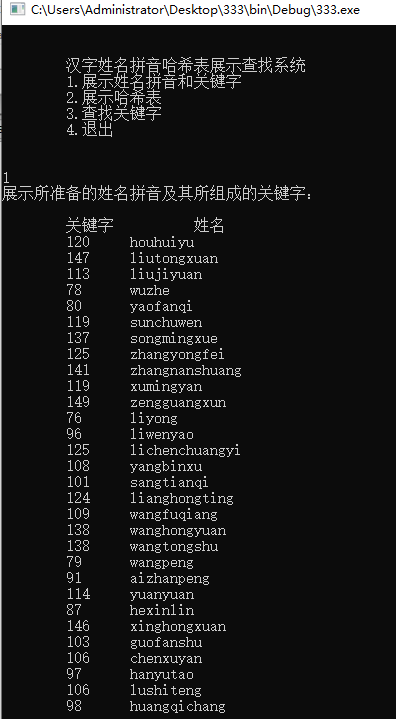##### 2）打印哈希表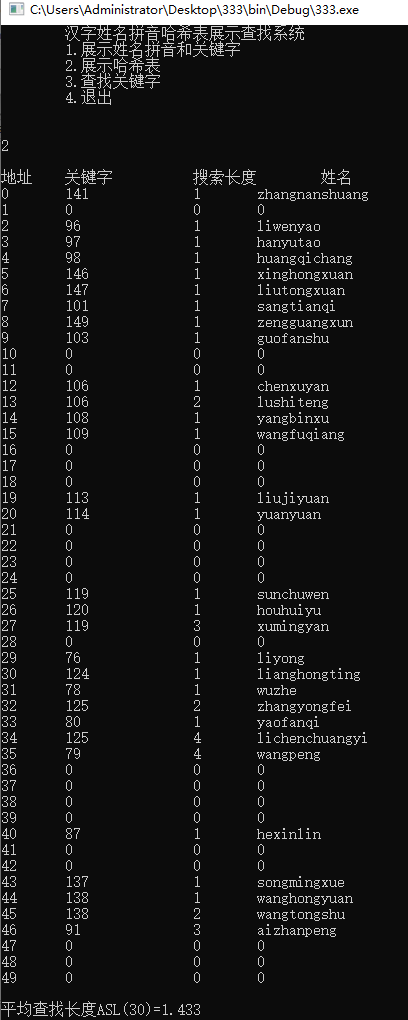##### 3）查找姓名：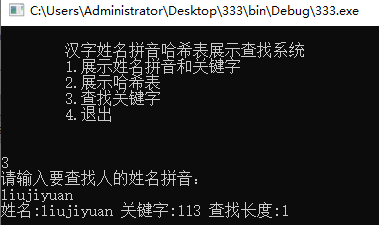## 7 结果分析

时间复杂度在没有冲突时是O(1)，实际上要根据冲突多少来确定，最差情况下会退化成O(n)。空间复杂度是O(n)。

展开全文数据结构 哈希表
• 根据数据结构知识，设计哈希表的相关内容 二、需求分析 [问题描述] 针对某个集体中人名设计一个哈希表，使得平均查找长度不超过 R，并完成相应的建表 和查表程序。 [基本要求] 假设人名为中国人姓名的汉语拼音形式。...

# 一、实验题目

根据数据结构知识，设计哈希表的相关内容

# 二、需求分析

[问题描述]
针对某个集体中人名设计一个哈希表，使得平均查找长度不超过 R，并完成相应的建表
和查表程序。
[基本要求]
假设人名为中国人姓名的汉语拼音形式。待填入哈希表的人名共有 30 个，取平均查找
长度的上限为 2。哈希函数用除留余数法构造，用线性探测再散列法或链地址法处理冲突。
[测试数据]
取读者周围较熟悉的 30 个人名。
[选作内容]
（1）从教科书上介绍的集中哈希函数构造方法中选出适用者并设计几个不同的哈希函
数，比较他们的地址冲突率（可以用更大的名字集合作实验）。
（2）研究这 30 个人名的特点，努力找一个哈希函数，使得对于不同的拼音名一定不发
生地址冲突。
（3）在哈希函数确定的前提下尝试各种不同处理冲突的方法，考察平均查找长度的变
化和造好的哈希表中关键字的聚集性。

# 三、概要设计

代码如下

/*pmj 20191220*/
#include<iostream>
#include<malloc.h>
#include<string.h>
using namespace std;
#define geshu 50                       //哈希表的长度
#define M 50
#define NAME_NO 30
typedef struct {
char *name;
int k;                                //拼音所对应的关键字
} Name;
typedef struct {
char *name;
int k;                                //拼音所对应的关键字
int len;                               //查找长度
} HASH;
Name ren[geshu];
HASH halt[geshu];
//main函数
int main() {
char *z;
int r, s0;
float average = 0;
for(int i=0; i<geshu; i++) {
cin>>ren[i].name;
}
for (int i = 0; i < NAME_NO; i++) {               //求整数
s0 = 0;
z = ren[i].name;                       //方法：将字符串的各个字符所对应的ASCII码相加，所得的整数做为哈希表的关键字
for (r = 0; *(z + r) != '\0'; r++)          //当字符为空时停止计算
s0 = *(z + r) + s0;
ren[i].k = s0;                         //利用循环将所有字符的ASCII码值相加
}                                               //最终的到的值就是ASCII码值和
for (int i = 0; i < geshu; i++) {               //哈希表的初始化
halt[i].name = "";
halt[i].k = 0;
halt[i].len = 0;
}
for (int i = 0; i < NAME_NO; i++) {
int sum = 0;
int adr = (ren[i].k) % M;               //利用除留余数法构造哈希函数
int d = adr;
if (halt[adr].len == 0) {                 //如果不冲突则存储到哈希表中
} else {                                     //存在冲突
do {
d = (d + ((ren[i].k)) % 10 + 1) % M;
sum = sum + 1;                       //查找次数加1
} while (halt[d].k != 0);
halt[d].k = ren[i].k;
halt[d].name = ren[i].name;     //数值存入哈希表
halt[d].len = sum + 1;                //接着查找下一个字符
}
}
cout << "姓名为：" <<"\t" << "地址为：" << "\t" << "关键字为：" << "\t\t" << "搜索的长度为：" << "\t" << "H为：" << "\t\t"<< endl;  //显示的格式
for (int i = 0; i < 50; i++) {
cout << halt[i].name<< i << "\t" << halt[i].k << "\t\t" << halt[i].len << "\t\t" << (halt[i].k) % M << "\t\t"  << endl;
}                                                 //依次输出
for (int i = 0; i < geshu; i++)
average += halt[i].len;                    //计算平均查找长度
average /= NAME_NO;
cout << "平均查找长度：ASL " << NAME_NO << "=" << average << endl;
}


# 四、调试分析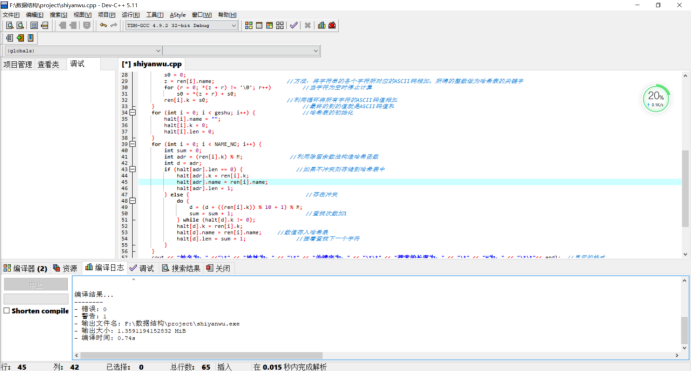调试结果如图所示，无错误。

# 五、使用说明

第一步：在程序中输入30个人名
第二步：输出地址、关键字、探索长度、H、姓名

# 六、测试结果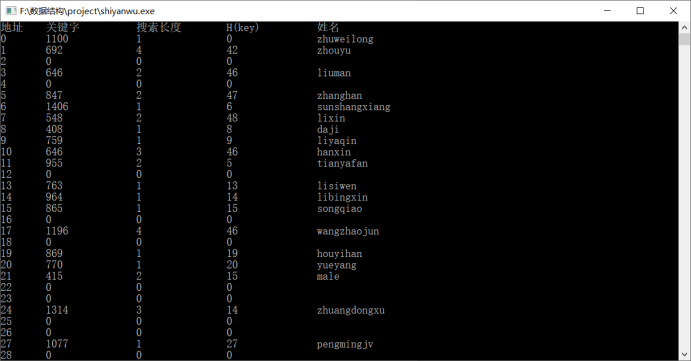# 七、其他数据结构实例

以上内容为个人学习总结，如有遗漏或者错误请在评论区中指正！！！

如果看完觉得有所收获的话，记得一键三连哦，谢谢大家！

展开全文数据结构 哈希表...